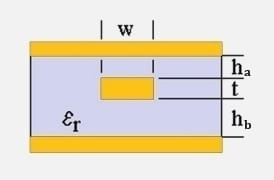Stripline Impedance

## Asymmetric Zo Calculator### Inputs

Trace Thickness
t
Height Above Trace
ha
Height Below Trace
hb
Trace Width
w
Substrate Dielectric
εr

### Output ⊕

Impedance (Zo):
76.6 Ω
Capacitance (C):
0.913 pF/cm
Propagation delay (Tpd):
69.9 ps/cm
Inductance (L):
5.36 nH/cm

View Notes

#### Calculation Notes

An asymmetric stripline is constructed between two plane layers, where the distance above the trace is not equal to the distance below.

Factors that influence the impedance calculation include:-

• Trace thickness (t)
• Height above trace (ha)
• Height below trace (hb)
• Trace width (w)
• Substrate dielectric (εr)

Z_{o}\approx \frac{80}{\sqrt{\varepsilon_{r}}}\times\ln\left(\frac{1.9\times(2h_{a}+t)}{(0.8w+t)}\right)\times \left(1-\frac{h_{a}}{4\times h_{b}}\right)

References:
• RF Design Guide Systems, Circuits and Equations (ISBN 0-89006-754-6)
• High-Speed Digital Design (ISBN 0-13-395724-1)
• Signal Integrity Issues and Printed Circuit Board Design (ISBN 0-13-335947-6)
• IPC-2141 Controlled Impedance Circuit Boards and High Speed Logic Design

Disclaimer: The information and this tool are provided with no liability of any kind whatsoever, use at your own risk.